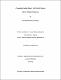## Computationally efficient methods for sparse tensor signal processing2021-12-31
##### Authors
Wickramasingha, Ishan
##### Abstract
Many state-of-the-art algorithms typically solve Tensor (multi-dimensional) problems using linear algebra by vectorizing tensor signals. However, the size of the tensors increases in polynomial order with the tensor order (dimensions); Therefore, higher-order tensor problems could be challenging to store or solve practically. This research aimed to develop novel tensor-based methods using multilinear algebra to solve tensor problems efficiently with significantly lower computational resources. Sparse signal representations, typically obtained by solving sparse least-squares(LS) problems, result in simpler and faster processing and lower memory storage requirements. However, solving a sparse LS problem for a large tensor signal is computationally infeasible. Therefore, we develop the Tensor Least Angle Regression (T-LARS) algorithm, a generalization of Least Angle Regression (LARS) to solve large L0 or L1 constrained sparse multilinear least-squares(MLS) problems efficiently for all critical values of the regularization parameter. Sparse weighted MLS, generalize the sparse MLS problem, where a weights matrix incorporates prior information about parameters and data. We generalized the T-LARS to develop the Weighted Tensor Least Angle Regression (WT-LARS), an efficient algorithm to solve L0 or L1 constrained weighted MLS problems. The T-LARS could not initialize with solutions outside of the Pareto curve because it will violate the optimality conditions of T-LARS. Therefore, we developed the Tensor Dynamic Least Angle Regression (TD-LARS) algorithm, a multilinear generalization of the L1-Homotopy algorithm to efficiently solve L1 constrained MLS problems using nonzero initial solutions located on or off of the Pareto curve. We introduced the Multilinear Elastic Net by generalizing the one-dimensional Elastic Net to solve strictly convex L1 and L2 constrained MLS problems, and we developed the Tensor Elastic Net (T-NET) algorithm to solve the Multilinear Elastic Net problems efficiently. We also developed the tensor task-driven dictionary learning (T-TDDL) framework by generalizing the one-dimensional task-driven dictionary learning (TDDL) that could work as an efficient online data-driven or task-driven dictionary learning framework for solving large multi-dimensional supervised, semi-supervised and unsupervised machine learning problems. Experimental results show the validity and performance of T-LARS, WT-LARS, TD-LARS, and T-NET in obtaining sparse multilinear representations of tensor signals and the performance of T-TDDL in multi-dimensional regression and classification tasks.
##### Keywords
Tensor Signal Processing, Sparse Signal Representations, Multilinear Least Squares, Tensor Least Angle Regression, Weighted Multilinear Least Squares, Multilinear Elastic Net, Online Tensor Task Driven Dictionary Learning
##### Citation
I. Wickramasingha, M. Sobhy, A. Elrewainy, and S. S. Sherif, “Tensor least angle regression for sparse representations of multi-dimensional signals,” Neural Comput., vol. 32, no. 9, pp. 1697–1732, Sep. 2020,https://doi.org/10.1162/neco_a_01304.